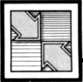`COMPUTE! ISSUE 64 / SEPTEMBER 1985 / PAGE 10`Readers Feedback  The Editors and Readers of COMPUTE! Relational Operators I recently typed in the TI-99/4A game "Circus" (COMPUTE!, February 1984) and noticed the following statement in line 50:  SC=SC+(H=120)*-50+(H=112)*-7  5+(H=104)*-100+((H=128)*(M1=  1)*250) How does this statement work? Dan Schwarz Although your question concerns a TI program, the answer applies to BASIC programming on a wide variety of computers. The complex statement that has you puzzled calculates the game score (variable SC) by using the equal sign (=) as a relational operator. Though its syntax looks odd, it efficiently takes the place of several IF-THEN statements.     In "Circus" the balloon (variable H) popped by the clown can be in the bottom row (character number 120), in the middle row (character 112), or the top row (104). Character 128 signifies the bonus balloon. A bottom row balloon scores 50 points, the middle row scores 75, the top row is worth 100, and a bonus balloon scores 250 points provided its color is yellow (M1=1; see line 80 of the program).     The expression (H=120) doesn't change the value of H. Instead, it performs a logical test similar to IF. When H equals 120-when you pop a bottom-row balloon-this expression returns a value of -1. Any expression that evaluates to -1 is considered to be true. When H equals any other number, the computer returns 0 to show the expression is false. (TI, Commodore, and IBM PC/PCjr computers evaluate true expressions to -1; Apple, Atari, and Timex/Sinclair computers use 1 rather than -1.)     Say that the clown pops a balloon in the bottom row. Since H equals 120, the expression (H=120) is true and evaluates to -1. This value is multiplied by -50 to add 50 to the score (multiplying two negative numbers produces a positive number). Since H=120 is true, the other expressions (H=112, H=104, and H=128) are false, so the multiplications yield 0 and the score doesn't change. The remaining expressions in the example increment the score when you pop balloons in the middle and upper rows or pop the bonus balloon (character 128) when it's yellow. Other relational operators include <, >, AND, OR, and NOT (if available in your dialect of BASIC). String expressions work as well as numeric expressions, and relational operations are particularly efficient when combined with ON-GOTO or ON-GOSUB statements.